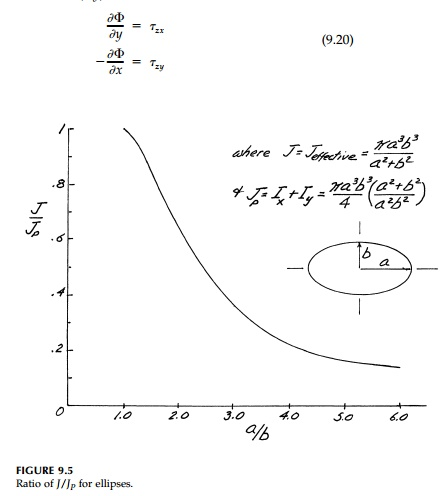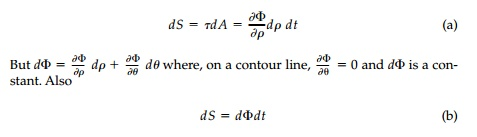Home | | Mechanics of Solids | PrandtlŌĆÖs Stress Function

# PrandtlŌĆÖs Stress Function

An alternative solution procedure that leads to a much simpler boundary condition, but a somewhat more difficult field equation.

PrandtlŌĆÖs Stress Function

An alternative solution procedure that leads to a much simpler boundary condition, but a somewhat more difficult field equation, involves the intro-duction of a stress function ŽĢ(x,y) defined as:By this definition, the equilibrium Equation (9.10) is automatically solved and the boundary condition Equation (9.15) becomes:As noted in previews, this is a Poisson equation for which a solution can always be found and the boundary condition

is particularly nice.

However, the power of the Prandtl stress function approach is in visualiz-ing torsional shear fields. It is easy to show that the stress function ŽĢ will transform in any direction as an invariant scalar function, and therefore in a new coordinate orientation x', y'Thus at any point the shear stress in one direction is equal in magnitude to the slope of the  ŽĢ surface in the perpendicular direction, and the maximum shear is the maximum slope of the stress function curve and acts tangent (along a contour) to that curve. If we visualize the stress function plotted in the z direction above the cross-section, then contours (of equal ŽĢ) can be plot-ted and:

a.  The shear Žä acts along these contours and is proportional to the shortest distance (slope normal) to the next contour;

b. Žämax occurs where the stress-hill contours become closest togeth-er, which will be on the boundary of the cross-section (generally where r = Rt(x2+y2) is a minimum.

From Figure 9.6 it is easy to show that the twisting moment Mt is propor-tional to the volume of this stress-function hill. The shear force dS on a differ ential element E will beTherefore, between two contour lines the resisting momentwhich is twice the volume of the stress-function hill.For example, consider the elliptic cross-section discussed previously. Assume a stress functionwhich must satisfy Equation (9.22), and the boundary condition ŽĢedge = 0. The volume of the stress-function hill (a paraboloid) is 0.5 ŽĆ abh and therefore:which corresponds to the result obtained by the direct St. Venant approach* using the warping function.

Study Material, Lecturing Notes, Assignment, Reference, Wiki description explanation, brief detail
Civil : Principles of Solid Mechanics : Torsion : PrandtlŌĆÖs Stress Function |

Related Topics